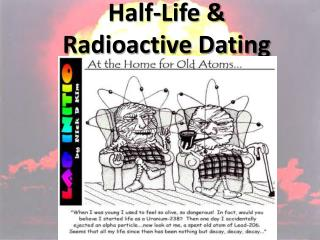DownloadDownload PresentationDownload Presentation- - - - - - - - - - - - - - - - - - - - - - - - - - - E N D - - - - - - - - - - - - - - - - - - - - - - - - - - -
##### Presentation Transcript

2. Half-Life: * the time it takes for ½ of the radioactive material to decay

3. Half-Life: * Table T Number of Half-life Total Half-lives Time time X =

4. Learning Check: A sample of a radioactive substance with an original mass of 16g was studied for 8 hours. When the study was completed, only 4g of the substance remained. What is the half-life? Step 1: 4g 16g Step 2: 1 4 Step 3: number of half- lifes (n) = 2 Step 4: t T = 1 4 1 n 2 = # of ½ lifes = 8 T = 2 T = 4 hrs

5. Learning Check: How much time must elapse before 16g of potassium-42 decays, leaving 2g of the original isotope? a. 8 X 12.4 hours c. 3 X 12.4 hours b. 2 X 12.4 hours d. 4 X 12.4 hours Answer is: C (3 half-lifes X 12.4 hours)

6. Learning Check: Based on Reference Table N, what fraction of a sample of gold-198 remains radioactive after 2.69 days? a. ¼ c. ¾ b. ½ d. 7/8 Answer is: b (1/2)

7. Learning Check: What is the half-life of sodium-25 if 1.00g of a 16g sample of sodium-25 remains unchanged after 237 seconds? a. 47.4 s c. 79.0 s b. 59.3 s d. 118 s Answer is: b (59.3 s) 4 half lifes = 237 T

8. Learning Check: After 32 days, 5 milligrams of an 80 milligram sample of a radioactive isotope remains unchanged. What is the half-life of this element? a. 8 days c. 16 days b. 2 days d. 4 days Answer is: a (8 days) 4 = 32 T

9. Learning Check: If 1/8 of an original sample of krypton-74 remains unchanged after 34.5 minutes, what is the half-life of krypton-74? a. 11.5 min c. 34.5 min b. 23.0 min d. 46.0 min Answer is: a (11.5 min) 3 = 34.5 T

10. Learning Check: How many days are required for 200.g of radon-222 to decay to 50.0g? a. 1.91 days c. 7.64 days b. 3.82 days d. 11.5 days Answer is: c (7.64) ½ life of Rn is 3.82 days 200g  50g = 2 half-lifes

11. Radioactive Decay Series: * The changes needed to become stable Takes 5.5 X 109 yrs

12. Radioactive Dating: * Determining age using half-life * For rocks use 238U * For things fairly recent use 14C